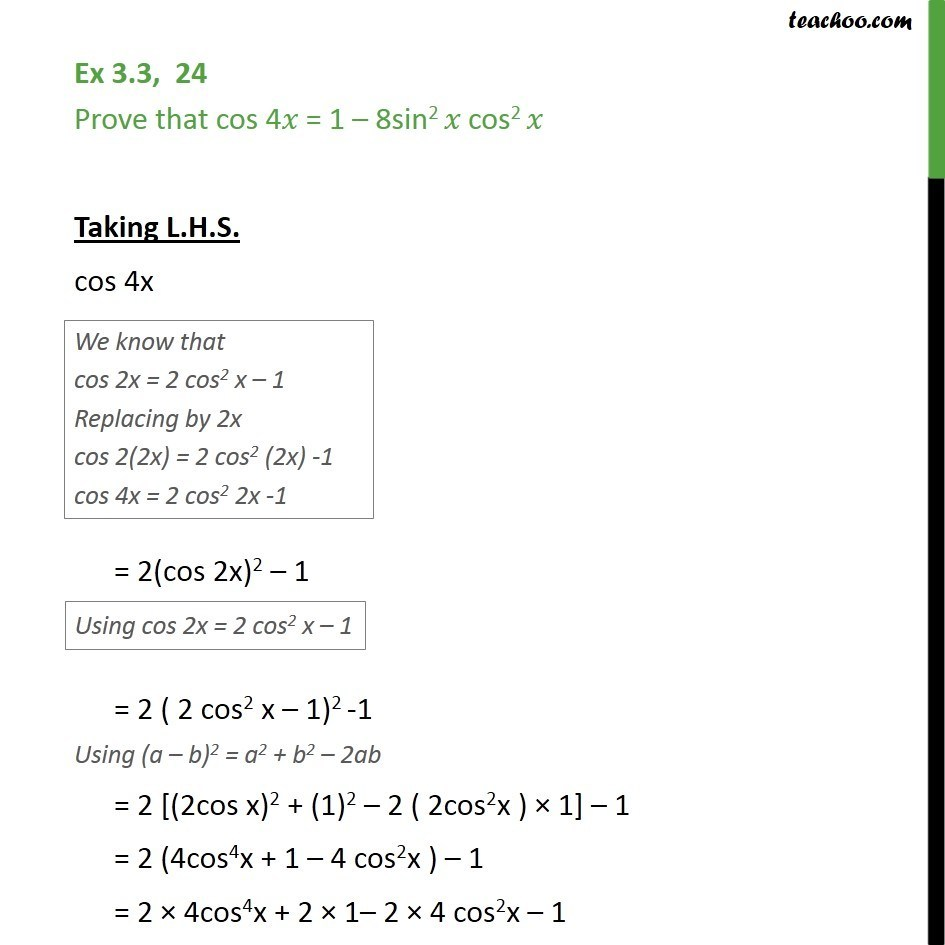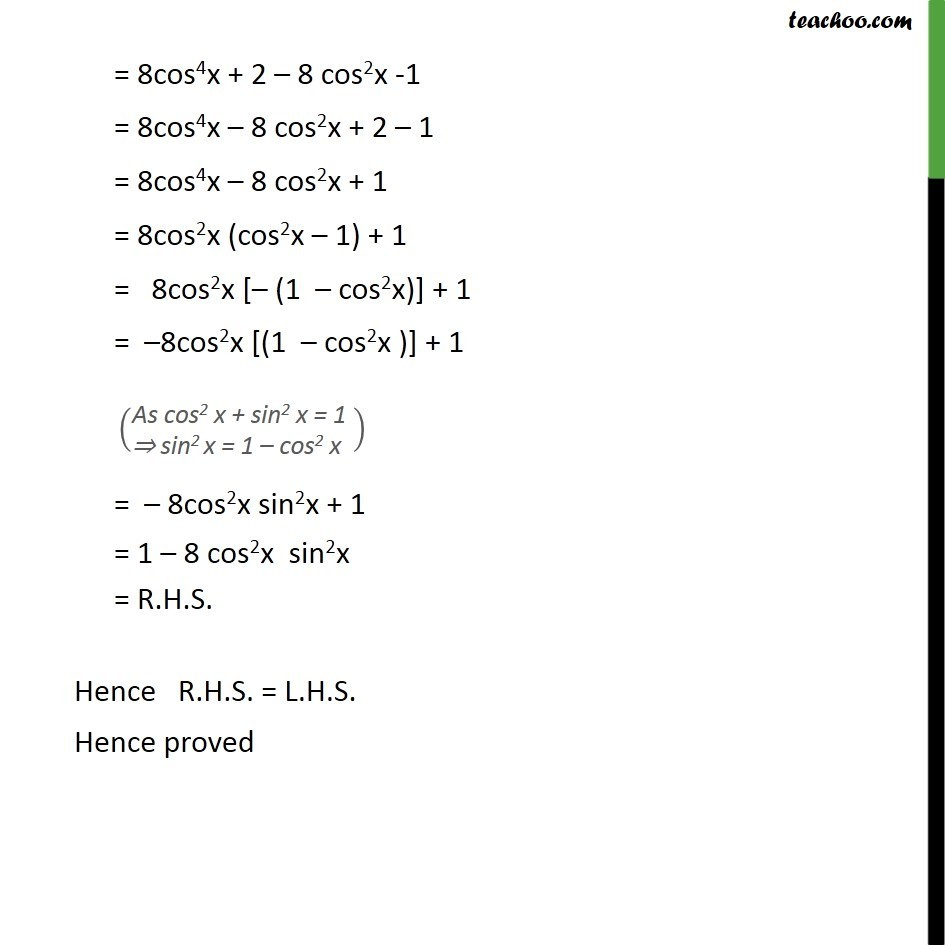Subscribe to our Youtube Channel - https://you.tube/teachoo

1. Chapter 3 Class 11 Trigonometric Functions
2. Concept wise
3. 2x 3x formula - Proving

Transcript

Ex 3.3, 24 Prove that cos 4𝑥 = 1 – 8sin2 𝑥 cos2 𝑥 Taking L.H.S. cos 4x = 2(cos 2x)2 – 1 = 2 ( 2 cos2 x – 1)2 -1 Using (a – b)2 = a2 + b2 – 2ab = 2 [(2cos x)2 + (1)2 – 2 ( 2cos2x ) × 1] – 1 = 2 (4cos4x + 1 – 4 cos2x ) – 1 = 2 × 4cos4x + 2 × 1– 2 × 4 cos2x – 1 = 8cos4x + 2 – 8 cos2x -1 = 8cos4x – 8 cos2x + 2 – 1 = 8cos4x – 8 cos2x + 1 = 8cos2x (cos2x – 1) + 1 = 8cos2x [– (1 – cos2x)] + 1 = –8cos2x [(1 – cos2x )] + 1 = – 8cos2x sin2x + 1 = 1 – 8 cos2x sin2x = R.H.S. Hence R.H.S. = L.H.S. Hence proved

2x 3x formula - Proving